# NCERT Exemplar - Surface Area and Volumes Class 10 Notes | EduRev

## Class 10 : NCERT Exemplar - Surface Area and Volumes Class 10 Notes | EduRev

The document NCERT Exemplar - Surface Area and Volumes Class 10 Notes | EduRev is a part of the Class 10 Course Mathematics (Maths) Class 10.
All you need of Class 10 at this link: Class 10

Q.1. A solid cylinder of radius r and height h is placed over other cylinder of same height and radius. The total surface area of the shape so formed is 4πrh + 4πr2.
Ans.
False.
Total surface area of the figure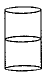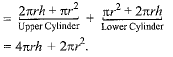Q.2. A rocket is in the form of a right circular cylinder closed at the lower end and surmounted by a cone with the same radius as that of the cylinder. The diameter and height of the cylinder are 6 cm and 12 cm, respectively. If the slant height of the conical portion is 5 cm, find the total surface area and volume of the rocket [Use π = 3.14].
Ans.
Here, r = 3 cm, h = 12 cm and l = 5 cm.
Total surface area of rocket
= C.S.A. of cone + C.S.A. of cylinder + area of base
= πrl + 2 πrh + πr2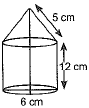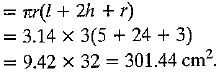Volume of the rocket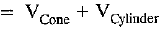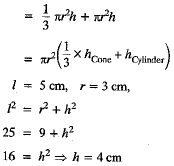Volume of the rocket =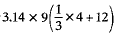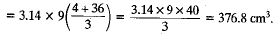Offer running on EduRev: Apply code STAYHOME200 to get INR 200 off on our premium plan EduRev Infinity!

## Mathematics (Maths) Class 10

165 videos|384 docs|103 tests

,

,

,

,

,

,

,

,

,

,

,

,

,

,

,

,

,

,

,

,

,

;